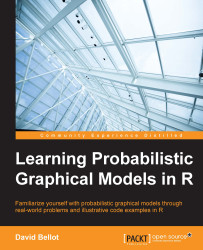•#### Learning Probabilistic Graphical Models in R#### Overview of this book

Probabilistic graphical models (PGM, also known as graphical models) are a marriage between probability theory and graph theory. Generally, PGMs use a graph-based representation. Two branches of graphical representations of distributions are commonly used, namely Bayesian networks and Markov networks. R has many packages to implement graphical models. We’ll start by showing you how to transform a classical statistical model into a modern PGM and then look at how to do exact inference in graphical models. Proceeding, we’ll introduce you to many modern R packages that will help you to perform inference on the models. We will then run a Bayesian linear regression and you’ll see the advantage of going probabilistic when you want to do prediction. Next, you’ll master using R packages and implementing its techniques. Finally, you’ll be presented with machine learning applications that have a direct impact in many fields. Here, we’ll cover clustering and the discovery of hidden information in big data, as well as two important methods, PCA and ICA, to reduce the size of big problems.
Learning Probabilistic Graphical Models in RCreditswww.PacktPub.comPrefaceFree Chapter
Probabilistic ReasoningExact InferenceLearning ParametersBayesian Modeling – Basic ModelsApproximate InferenceBayesian Modeling – Linear ModelsProbabilistic Mixture ModelsAppendixIndex## Examples of probabilistic graphical models

In this last section we will show several examples of PGM that are good candidates for exact inference. The goal of this section is to show realistic yet simple examples of what can be done and to provide the reader with ideas for developing his or her own models.

### The sprinkler example

This is an historical example which has been used in many textbooks. It is rather simple and shows a simple reasoning.

Let's say we look at our garden and see the grass is wet. We want to know why the grass is wet. There are two possibilities: either it was raining before or we forgot to turn off the sprinkler. Moreover, we can observe the sky. If it's cloudy, chances are it was raining before. However, if it was cloudy then presumably we didn't turn on the sprinkler, so it is more likely, in this case, we would have not forgotten to turn off the sprinkler.

This is a little example of causal reasoning that can be represented by a PGM. We identify four random variables...